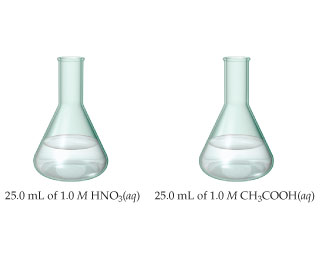# Problem: The samples of nitric and acetic acid shown here are both titrated with a 0.100 M solution of NaOH(aq).Determine whether each of the following statements concerning these titrations is true or false.A larger volume of NaOH(aq) is needed to reach the equivalence point in the titration of HNO3.

###### FREE Expert Solution

We are asked to determine whether the following statement concerning the given titration is true or false.

A larger volume of NaOH(aq) is needed to reach the equivalence point in the titration of HNO3.

1. Titration of 25.0 mL of 1.0 M HNO3 with 0.100 M NaOH solution

The reaction for this titration can be represented by:

HNO3 + NaOH → NaNO3 + H2O

In the reaction HNO3, a strong acid, will react with NaOH, a strong base to form a neutral solution.

At the equivalence point of the reaction, hydronium (H+) and hydroxide (OH-) ions will react to form water, leading to a pH of 7.

This is true for all strong acid and strong base reactions.

Recall that at the equivalence point of a titration:###### Problem Details

The samples of nitric and acetic acid shown here are both titrated with a 0.100 M solution of NaOH(aq).
Determine whether each of the following statements concerning these titrations is true or false.A larger volume of NaOH(aq) is needed to reach the equivalence point in the titration of HNO3.